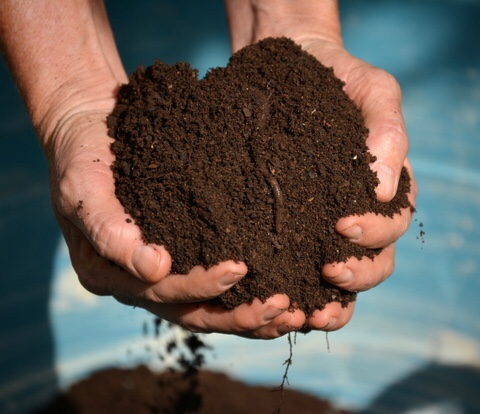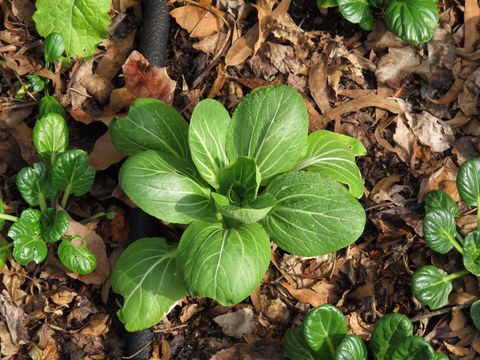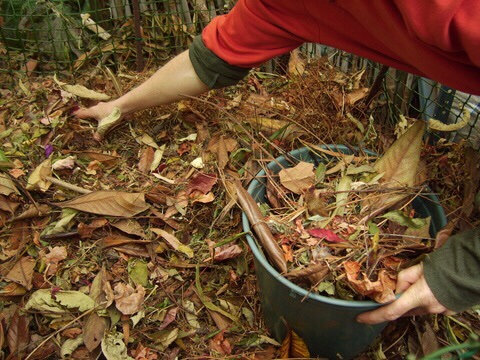# Jane's Delicious Garden Blog

## Calculating mulch.

Posted in Garden Diary by Jane Griffiths on the January 20th, 2020People often ask me how much mulch to add. It depends on what mulch you are using. Straw or leaves can be added a bit more thickly than compost for example, as they are looser.If you are buying mulch in bulk and want to work out how many cubic meters you need here is the process:

1. First work out the square meterage by multiplying the length by the width of each your beds.

2. Then combine these to get a total.

3. If you have circular beds the maths is a bit more complicated:

• Measure the radius of the circle (the distance from the center of the bed to the outer edge.)
• Then multiply that number by itself. Then multiply the total by 3.14 (π):

R x R x 3.14 = square meterage of a circle.

4. If your beds are odd shapes, divide them into imaginary rectangles and circles and add up the totals.

Now decide how deep the mulch will be. If I’m using compost and leaves mixed together I make it about 10cm but with straw it can be 10-15cm.

Take your total square meterage (meters) and multiply it by the thickness (centimetres). Divide it by 100 to get the metre cubed amount you need to buy.

For example:

40m² x 10cm deep = 400cm³

400cm³ ÷ 100 = 4m³

If you are buying in bags:

Compost is sold in 30dm³. This is 30 cubic decimetres and 10 bags make up one cubic meter.

For 40²m you would need 40 bags.